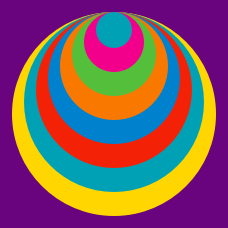Geometry

# 3D Composite Figures Warmup

The cube shown measures 6 units on each side. How many cubes with a side length of 2 units will fit inside?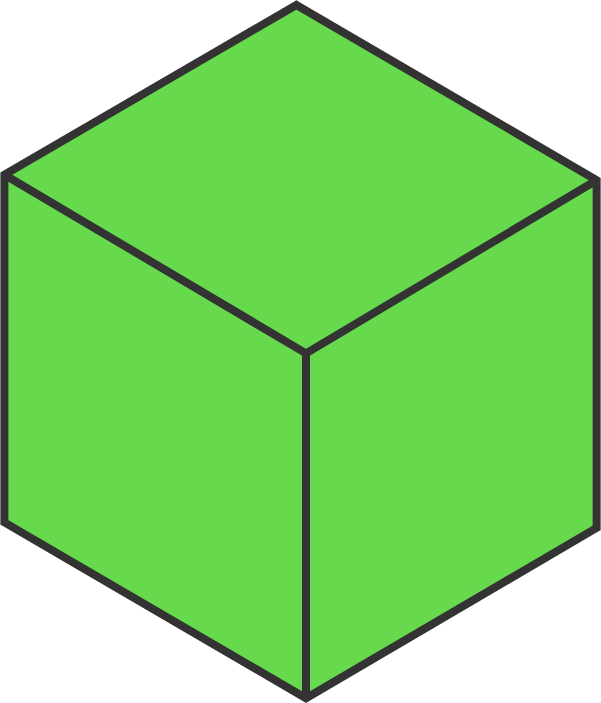Eight small unit cubes are stacked as shown. If one more cube is added on top, by how much will the surface area of the figure increase?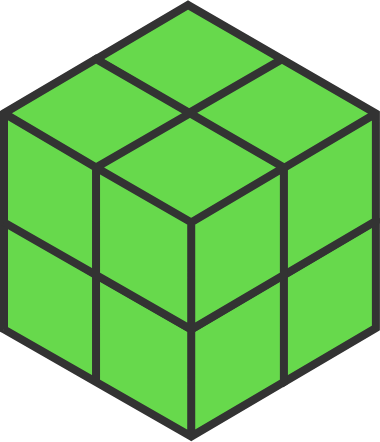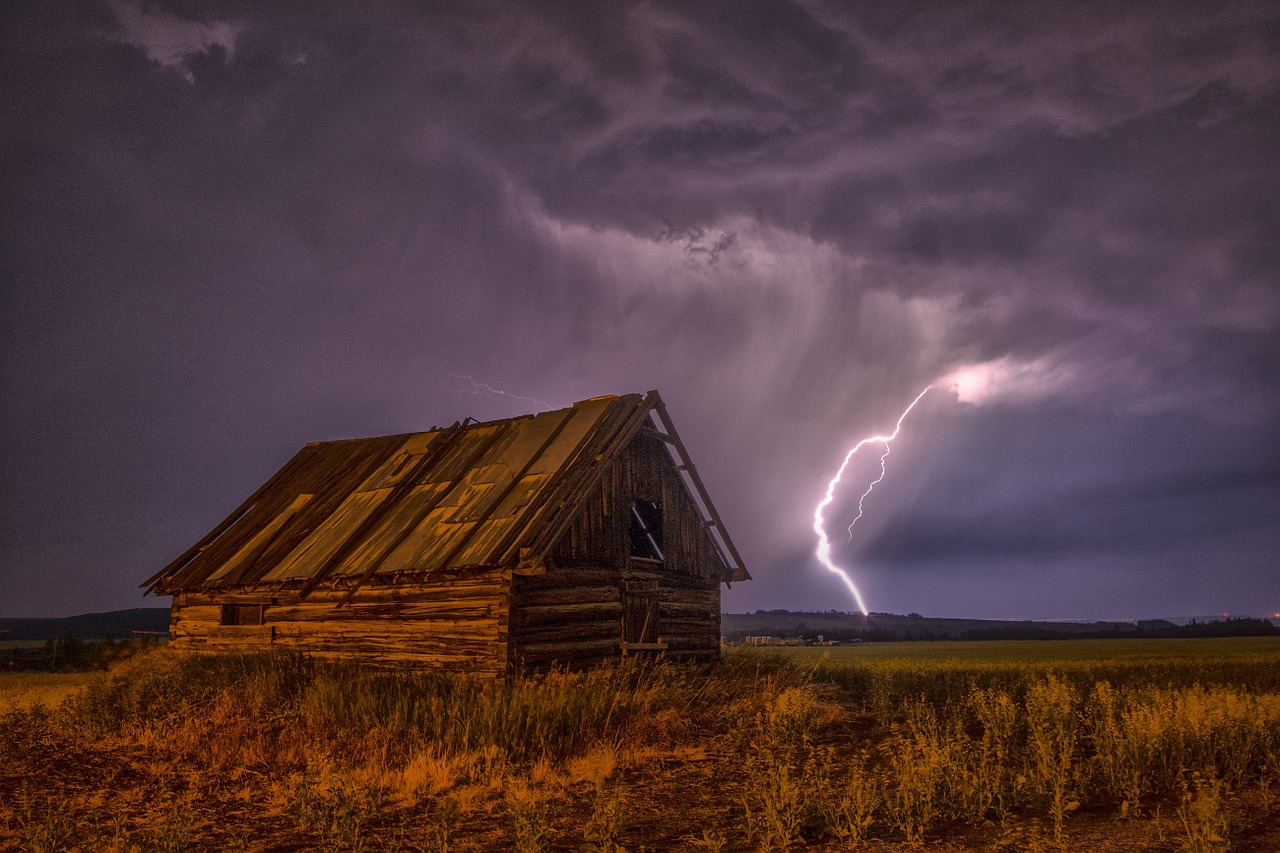To find the volume of the barn shown, Joy imagines breaking the barn into two pieces: a rectangular prism, or box, for the bottom and a triangular prism for the top. She will find the volume of each and add them together to find the total volume. Will her strategy work?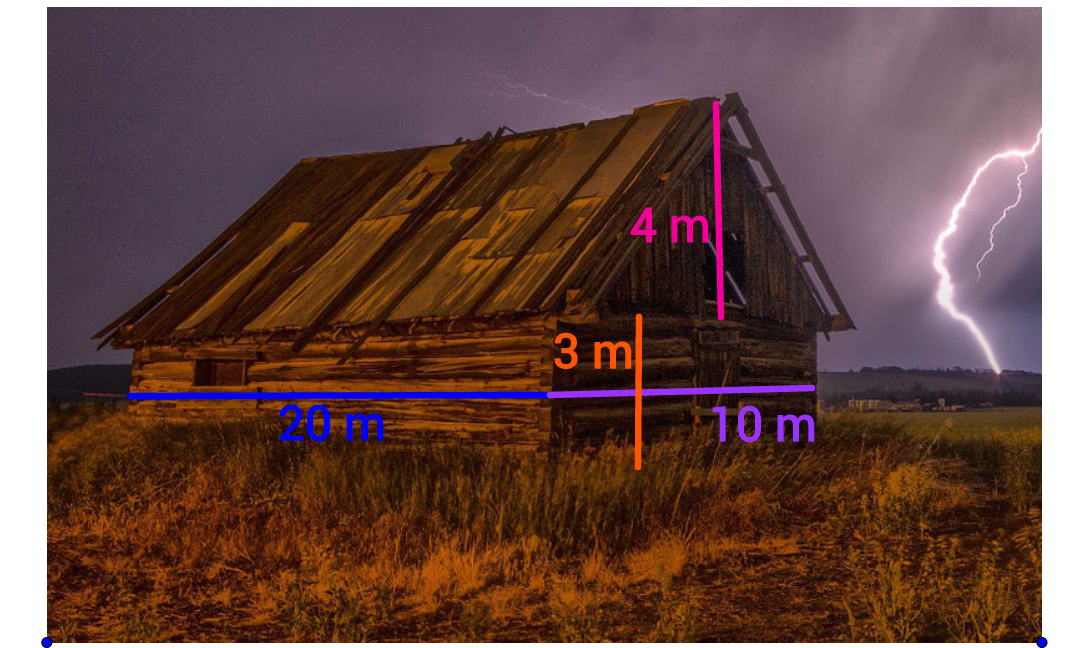Zach wants finding the volume of the barn by imagining that it is a box. He will find the volume of this entire box and subtract out the volume of the two triangular prism sections next to the roof that do not exist. Which expression could represent his thinking?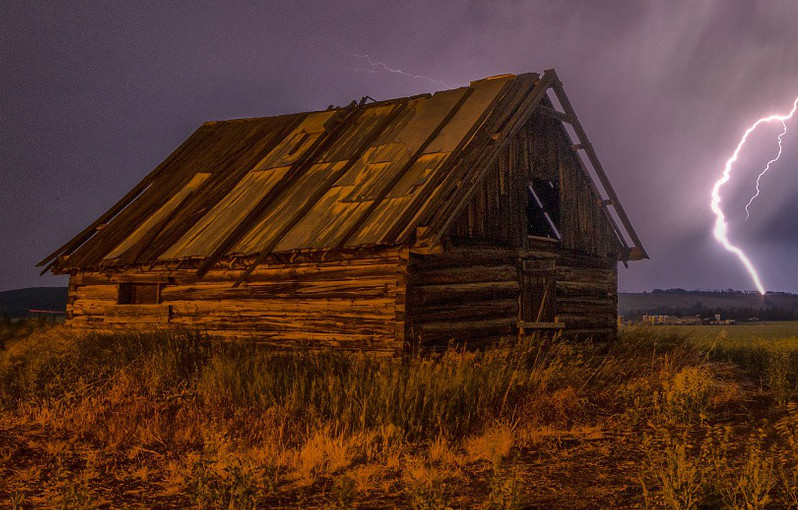True or False?

To estimate the external surface area of the barn, we could add together the area of six rectangles and two triangles.

(Note the floor is not considered external.)

×